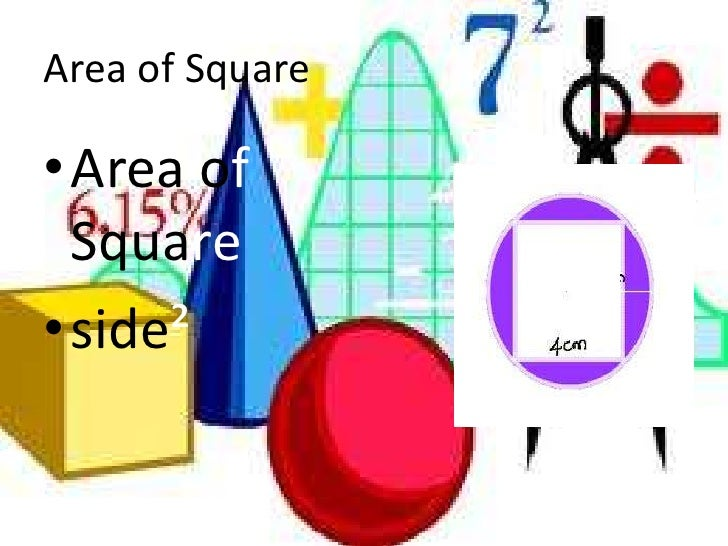# Quant Quiz on “MENSURATION” for SSC CGL 2016Q1. What will be the surface area of the sphere having 4 cm radius?
(a) 64 π sq cm
(b) 69 π sq cm
(c) 32 π sq cm
(d) 35 π sq cm

Q2. What is the diameter of the largest circle lying on the surface of a sphere of surface area 616 sq cm?
(a) 14 cm
(b) 10.5 cm
(c) 7 cm
(d) 3.5 cm

Q3. The diameter of the Moon is approximately one-fourth of the diameter of the Earth. What is the ratio (approximate) of their volumes?
(a) 4 : 16
(b) 1 : 64
(c) 1 : 4
(d) 1 : 128

Q4. A sphere and a hemisphere have the same surface area. The ratio of their volumes is :
(a) √3/4 ∶1
(b) (3√3)/4 ∶1
(c) √3/8 ∶1
(d) none of these

Q5. A hemisphere has 3 cm radius. Calculate its volume.
(a) 4/3  π cm^3
(b) 13 π cm^3
(c) 18 π cm^3
(d) 19/π  cm^3

Q6. A hemispherical bowl has 3.5 cm radius. It is to be painted inside as well as outside. Find the cost of painting it at the rate of Rs. 5 per 10 sq cm.
(a) Rs. 50
(b) Rs. 81
(c) Rs. 56
(d) Rs. 77

Q7. If the surface area of a sphere is 616 sq cm, what is its volume?
(a) 4312/3 cm^3
(b) 4102/3 cm^3
(c) 1257 cm^3
(d) 1023 cm^3

Q8. If the ratio of the diameters of two spheres is 3 : 5, then what is the ratio of their surface areas?
(a) 9 : 25
(b) 9 : 10
(c) 3 : 5
(d) 27 : 125

Q9. If 64 identical small spheres are made out of a big sphere of diameter 8 cm, what is surface area of each small sphere?
(a) π cm^2
(b) 2 π cm^2
(c) 4 π cm^2
(d) 8 π cm^2

Q10. A hemisphere has 28 cm diameter. Find its curved surface area.
(a) 1232 sq cm
(b) 1236 sq cm
(c) 1238 sq cm
(d) 1233 sq cm

Q11. A metallic sphere of radius 12 cm is melted into three smaller spheres. If the radii of two smaller spheres are 6 cm and 8 cm, the radius of the third is:
(a) 14 cm
(b) 16 cm
(c) 10 cm
(d) 12 cm

Q12. If the radius of a sphere is increased by 3%, then what per cent increase takes place in surface area of the sphere?
(a) 6.09%
(b) 7%
(c) 5.06%
(d) 9%

Q13. If radius of a sphere is decreased by 24%, by what per cent does its surface area decrease?
(a) 44%
(b) 49%
(c) 42.24%
(d) 46.2%

Q14. If the radius of a sphere is increased by 100%, by what per cent does its volume increase?
(a) 300%
(b) 900%
(c) 500%
(d) 700%

Q15. Weight of a solid metallic sphere of radius 4 cm is 4 kg. The weight of a hollow sphere made with same metal, whose outer diameter is 16 cm and inner diameter is 12 cm, is
(a) 20.5 kg
(b) 15.5 kg
(c) 16.5 kg
(d) 18.5 kg

×

## Download success!

Thanks for downloading the guide. For similar guides, free study material, quizzes, videos and job alerts you can download the Adda247 app from play store.

Thank You, Your details have been submitted we will get back to you.

## Leave a comment

×
Login
OR

Forgot Password?

×
Sign Up
ORForgot Password
Enter the email address associated with your account, and we'll email you an OTP to verify it's you.Reset Password
Please enter the OTP sent to
/6

×
CHANGE PASSWORD